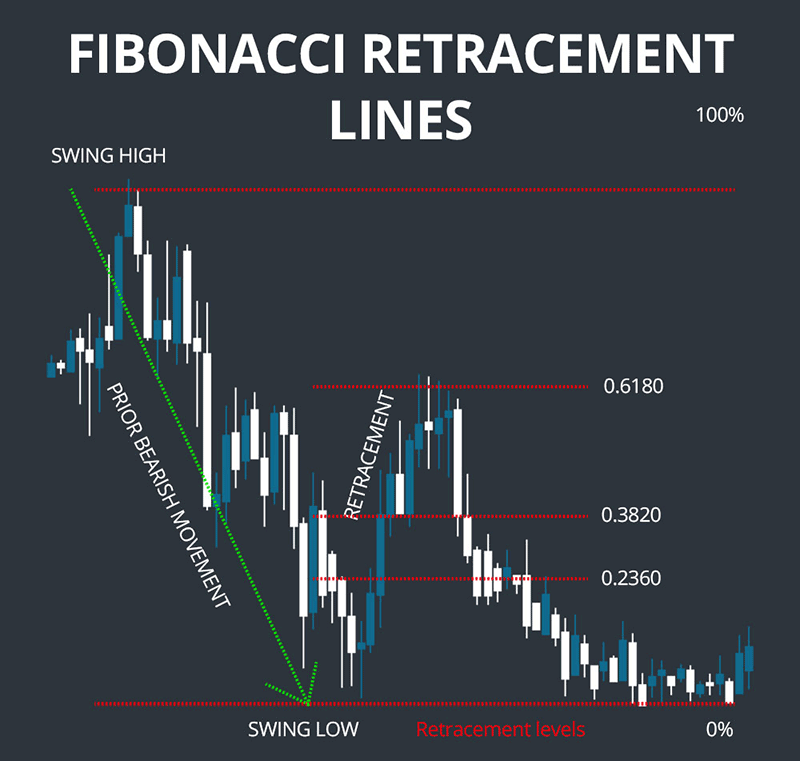'

Search
GBP
ENG

# Fibonacci Retracement - Advanced Techniques

When used in the Forex markets Fibonacci Retracements are ratios that help identify potential reversal levels and show which way the market may go next, these ratios are found in the Fibonacci sequence. The most popular Fibonacci Retracement levels that are used for trading are 61.8% and 38.2%, it may be worth noting that 38.2% is often rounded to 38% and 61.8 is rounded to 62%. The Fibonacci retracement levels use lines to show where the possible support and resistance levels are located. The actual percentage values show how much of a previous price move has retraced.

If you are new to Fibonacci numbers then you may want to visit the website below which will get you up to speed.